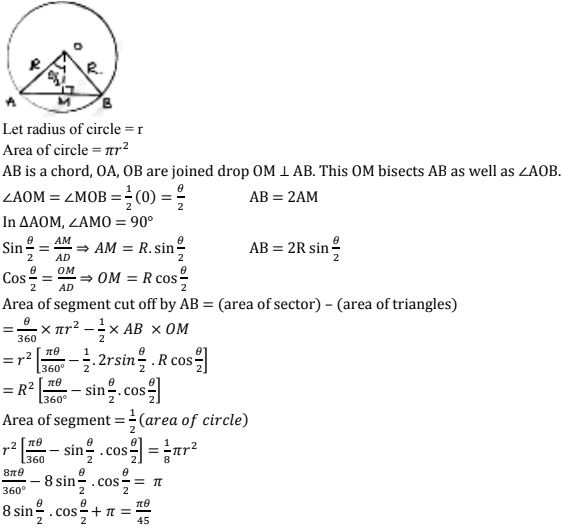# Chapter 15 Areas Related to Circles RD Sharma Solutions Exercise 15.3 Class 10 Maths

 Chapter Name RD Sharma Chapter 15 Areas Related to Circles Book Name RD Sharma Mathematics for Class 10 Other Exercises Exercise 15.1Exercise 15.2Exercise 15.4 Related Study NCERT Solutions for Class 10 Maths

### Exercise 15.3 Solutions

1. AB is a chord of a circle with centre O and radius 4cm. AB is length 4cm and divides circle into two segments. Find the area of minor segment

Solution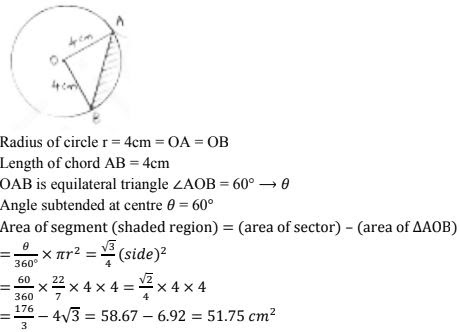2. A chord of circle of radius 14 cm makes a right angle at the centre. Find the areas of minor and major segments of the circle.

Solution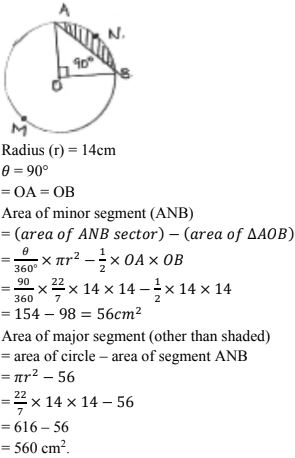3. A chord 10 cm long is drawn in a circle whose radius is 5√2 cm. Find the area of both segments

Solution

Given radius = r = 5√2 cm = OA = OB
Length of chord AB = 10 cm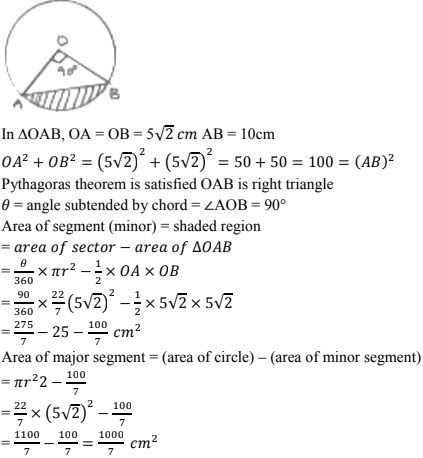4. A chord AB of circle, of radius 14 cm makes an angle of 60° at the centre. Find the area of minor segment of circle.

Solution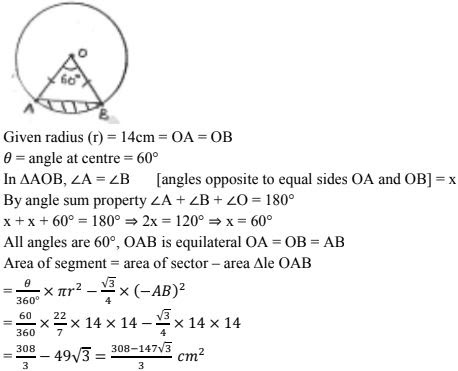5. AB is the diameter of a circle, centre O. C is a point on the circumference such that ∠COB = θ. The area of the minor segment cutoff by AC is equal to twice the area of sector BOC. Prove that sinθ/2 cosθ/2 = π(1/2 - θ/120°)
Solution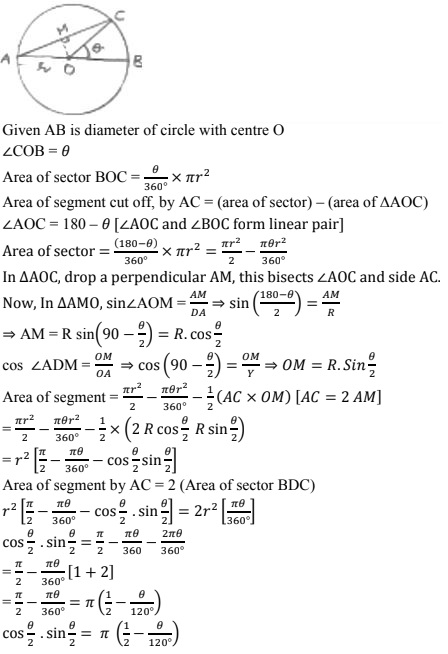6. A chord of a circle subtends an angle θ at the centre of circle. The area of the minor segment cut off by the chord is one eighth of the area of circle. Prove that 8 sinθ/2. cosθ/2
π = πθ/45
Solution